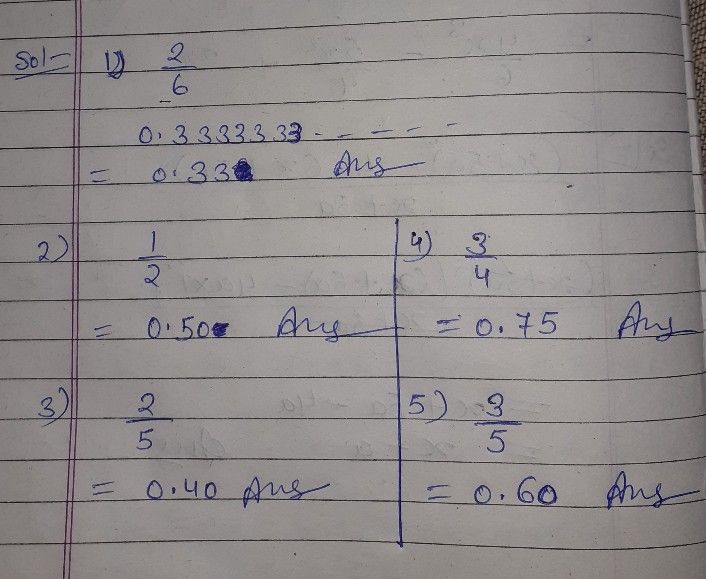Symbol
ProblemAdditional Activities $A$ A Express the Fractions to Decimals forms. Round off your answers to hundredths place. $1.\right)$ $\dfrac {2} {6}=$ $2.1$ $\dfrac {1} {2}=$ $3.1$ $\dfrac {2} {5}=$ $'3$ $x\times$ $S$ $'.3$ $s>$ $21$
7th-9th grade
Other
Question content
Can i ask help?
SolutionQanda teacher - SHIVKUMARCokk
I hope you understand
If you have any problem with answer or my hand writing plz ask me first or inform me first.
But If you're satisfied plz do like button and give 5 stars rating as a gift of Thank you...??Student
okay sirQanda teacher - SHIVKUMARC
plz evaluate this question
plz ..